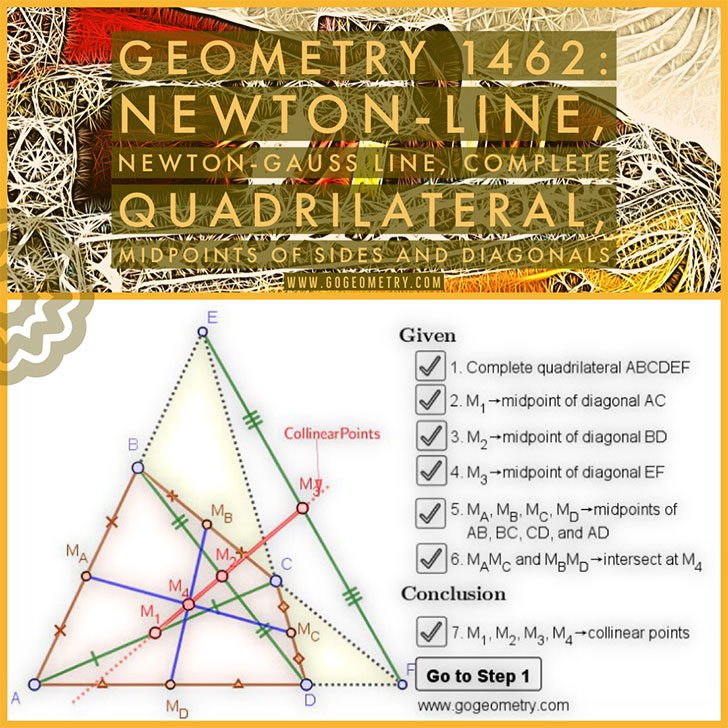# Dynamic Geometry 1462: Newton-Line, Newton-Gauss Line, Complete Quadrilateral, Midpoints of Sides and Diagonals

Given a complete quadrilateral ABDEF (see the figure below) M1, M2, and M3 are the midpoints of AC, BD, and EF, respectively. The line segments MAMC and MBMD that connect the midpoints of opposite sides AB with CD and BC with AD intersect at M4. Prove that M1,M2,M3, and M4 are collinear points.

### Newton Line

Newton line is the line that connects the midpoints of the two diagonals in a quadrilateral other than a parallelogram.

### Newton-Gauss line

The midpoints of the diagonals of a complete quadrilateral lie on a line called Newton-Gauss line.

Complete quadrilateral ABCDEF is the figure determined by four lines, no three of which are concurrent, and their six points of intersection A, B, C, D, E, and F.

### Midpoint

Midpoint of a segment: the point on a line segment dividing it into two segments of equal length.

## Static Diagram of Dynamic Geometry 1462: Newton-Gauss Line## Poster of the Newton Line and Newton-Gauss Line using iPad Apps### Classroom Resource:Interactive step-by-step animation using GeoGebra

This step-by-step interactive illustration was created with GeoGebra.

• To explore (show / hide): click/tap a check box.
• To stop/play the animation: click/tap the icon in the lower left corner.
• To go to first step: click/tap the "Go to step 1" button.
• To manipulate the interactive figure: click/tap and drag the blue points or figures.

GeoGebra is free and multi-platform dynamic mathematics software for all levels of education that joins geometry, algebra, tables, graphing, statistics and calculus application, intended for teachers and students. Many parts of GeoGebra have been ported to HTML5.